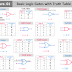Hey, in this article we are going to see the different types of logic gates with their truth table, boolean expression, and symbols. We know that Logic gates are the basic building blocks or primary structure of any digital electronic circuit. The digital electronic circuit cannot be built without logic gates. The logic gate itself is an electronic circuit built using switching components such as Transistors, Diodes, etc. Logic gates have one or multiple inputs and a single output. The output is based on the input at a certain logic.

Based on the core logic the most basic logic gates are AND, OR, and NOT. Further, they are specially designed or combined to make other gates such as NAND, NOR, XOR, XNOR, etc. In this article, we will see the truth table, symbol, and boolean expression of all the basic logic gates (two inputs) and three input logic gates.

## Basic Logic Gates with Truth Table, Symbol, and Boolean Expression

Here, you can see a total of eight(8) types of logic gates are shown.

### YES Gate

The output is similar to the input. That when the input is low the output will be low and when the input is high the output will be high.

### NOT Gate

The output is complementary to the input. That means when the input is low(0) the output will be high(1) and when the input is high(1) the output will be low(0).

### AND Gate

It executes the AND operation(multiplication). When both of the two inputs are high the output will be high otherwise all time the output will be low.

### NAND Gate

It is the combination of AND Gate and NOT Gate. When both of the two inputs are high the output will be low otherwise all time the output will be high.

### OR Gate

It executes the OR operation(Summation). When both of the two inputs are low the output will be low otherwise all time the output will be high.

### NOR Gate

It is the combination of OR gate and NOT Gate. When both of the two inputs are low the output will be high otherwise all time the output will be low.

### XOR Gate

It is also known as EX-OR gate or X-OR gate. When both of the two inputs are low or high the output will be low and when both inputs are different(a combination of high and low) the output will be high.

### XNOR Gate

It is the combination of XOR gate and NOT gate.  It is also known as X-NOR Gate or EX-NOR Gate. When both of the two inputs are low or high the output will be High and when both inputs are different(a combination of high and low) the output will be Low.

## Three(3) Input Logic Gates with Truth Table, Symbol, and Boolean Expression

Here, you can see the total of six(6) types of three input logic gates which are,
3 input AND gate, 3 input NAND gate, 3 input OR Gate, 3 input NOR gate, 3 input XOR gate, and 3 input XNOR gate.

Different Types of Logic Gates with Truth Table, ExpressionReviewed by Author on April 22, 2023 Rating: 5